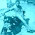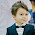## Saturday, July 18, 2015

### Dates and Calendars - Zeller's Rule Shortcut

Calculate the day of the week for any date. Zeller’s Rule can be used to find the day on any particular date in the calendar in the history. All you have to know is the formula given below and how to use it.

Zeller’s Rule Formula:
F = K + [(13xM - 1)/5] + D + [D/4] + [C/4] – 2C
where, K = Date, M = Month, C = The first two digits year and D = Last two digits of the year
* In Zellers rule, months start from March. March = 1, April = 2, May = 3 and so on… till Dec = 10,
Jan = 11 Feb. = 12
* Also remember that when you have to find day of the first or second month of any year, then Year=Given year-1 i.e., When you want to find Day of 15-2-1990., K=15, Month=12, D=Given Year-1=1990-1=1989=89

Ex: find the day of the 27-08-2014 ?
Sol: K=27,M=6,C=20 and D=14
Replacing the values in the formula, we get F = 27 + [{(13 x 6)- 1}/5] + 14 + 14/4 + 20/4 – (2 x 20)
Therefore, F = 27 + 77/5 + 14 + 14/4 + 20/4 –40
Which gives.. F =27 + 15.5 + 14 + 3.5 + 5 – 40
[ We have to Consider only the integral value and ignore the value after decimal. So, the equation changes a bit as shown below. We have just removed value after decimal ]
F = 27 + 15 + 14 + 3 + 5 – 40 Therefore, F = 3. Now that you have a numerical value for the day, divide the number by 7. We need the remainder only. For example, in this case, the remainder is 3.
Now, match the remainder with the chart below:
1 = Monday 2 = Tuesday 3 = Wednesday 4 = Thursday 5 = Friday 6 = Saturday 7 = Sunday
Here, 3 represents Wednesday .
So by Zeller’s rule, 27th of August, 2014 was on a Wednesday . So,Today is wednesday.
This formula will help you a lot in any Calendar question that you may encounter in Quant or DI. Remember that it is necessary to know the formula properly or else, even a little mistake can render the answer incorrect.
PS: It is natural for all of us to consider January as the first month of the year. However, we request you to please note that March should be treated as first month for using this formula.
With this, you are all set to rock any calendar question that comes in front of you.
The most important thing in this concept is to remember the formula as an incorrect interpretation can lead to a false answer.
Above zeller rule is applicable to find exact day of given date. If you to find the day of the given date in a problem where a day is given on a specified date. then we have to follow the given below procedure:

MODULE OBJECTIVE:
The module calendar is used to find many problems related to odd days, leap year, and counting of odd days and many. The module clock is used to find many problems related to find angle between hour and minute hand of a clock, at what time the hands of clock will be together and many.
We are supposed to find the day of the week on a given date. Odd Days:For this, we use the concept of 'odd days'. In a given period, the number of days more than the complete weeks are called odd days.
Leap Year:
(i). Every year divisible by 4 is a leap year, if it is not a century.
(ii). Every 4th century is a leap year and no other century is a leap year.
Note: A leap year has 366 days.
Examples:
i. Each of the years 1948, 2004, 1676 etc. is a leap year.
ii. Each of the years 400, 800, 1200, 1600, 2000 etc. is a leap year.
iii. None of the years 2001, 2002, 2003, 2005, 1800, 2100 is a leap year.
Ordinary Year: The year which is not a leap year is called an ordinary years. An ordinary year has 365 days.

Counting of Odd Days:
1.1 ordinary year = 365 days = (52 weeks + 1 day.)1 ordinary year has 1 odd day.
2.1 leap year = 366 days = (52 weeks + 2 days)
so,1 leap year has 2 odd days.
3.100 years = 76 ordinary years + 24 leap years= (76 x 1 + 24 x 2) odd days = 124 odd days.
= (17 weeks + days)5 odd days.
Number of odd days in 100 years = 5.
Number of odd days in 200 years = (5 x 2),3 odd days.
Number of odd days in 300 years = (5 x 3),1 odd day.
Number of odd days in 400 years = (5 x 4 + 1) 0 odd day.
Similarly, each one of 800 years, 1200 years, 1600 years, 2000 years etc. has 0 odd days.

Some codes to remember the months and weeks of number of odd days :
a) Week
Day             -       Code
Sunday       –         1
Monday      –         2
Tuesday      –         3
Wednesday –         4
Thursday    –         5
Friday         –         6
Saturday     –         0

b) Month
jan     –1
july    –0
feb     –4
Aug   –3
Mar   –4
Sep    –6
Apr    –0
Oct     –1
May   –2
Nov    –4
june    –5
Dec    –6

1.This comment has been removed by the author.

1.This comment has been removed by the author.

2.it's nice to hear from you
in the zellar rule
F=(value) is divided by 7
if you have got remainder as F=0 , 7 , 14..
then it is a sunday simply because any multiples of 7 is divisible by itself.
And in your case , you got F=0 or 7 or 14 and these are divisible by 7 .
I suppose you are able to understand what i have conveyed .

3.ok thanq..i got it..
what about if we get F=negative value..
for example,take 06-10-2002
and..
what about 06-04-2016???? i got F=-4
how can i divide this with 7? decimal values will come.please explain

2.Thanks for sharing informative Blog, its really precious for every one.
SSC Coaching in Indore